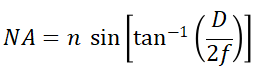# Numerical Aperture Calculators

This is an online calculator that calculates Numerical Aperture. Just enter the Refractive Index of the Core and Refractive Index of the Cladding to get the corresponding Numerical Aperture value.

### Result

• Numerical Aperture

Numerical Aperture is a dimensionless quantity that determines the ability of an optical fiber to gather light. It is the measure of the range of angles over which a system can accept light or the angular acceptance of a system for the incoming light. It is given by,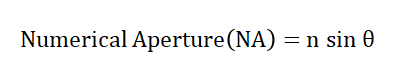Where 'n' is the refractive index of the medium from which the light enters a system. It is also a dimensionless quantity that describes the change in the velocity of light when travelling through that medium. Here 'θ' called the acceptance angle is the maximum incident angle at which an optical system will permit light into it.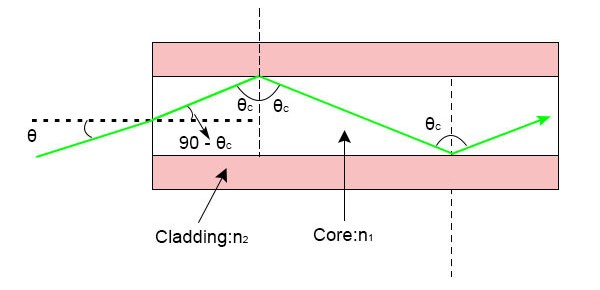In the case of a multimode fiber, the acceptance angle is determined by the refractive indices of the core, the cladding, and the medium in which the optical fiber is placed. In that case, we get the numerical aperture equation as,Where n1 and n2 are the refractive indices of the fiber core and cladding respectively.

Consider a ray of light is incident from a medium of refractive index n, at the acceptance angle into a fiber core of refractive index n1, applying snell’s law at the medium-core interface, we get,here θr is the angle of refraction for the incident ray.

From the figure,Here θc is known as the critical angle for total internal reflection.

Total internal reflection is an optical phenomenon in which waves arriving at the interface of two medias are not refracted to the second media, but is completely reflected back to the first media. It occurs when a wave travels from a denser medium to a rarer medium, i.e. the second medium has a lower refractive index when compared to the first medium. The minimum angle of incidence of an optical wave for which the total internal reflection occurs is known as the critical angle for that wave. It is given by,Now (1) becomes,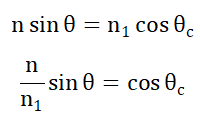Squaring on both sides, we get,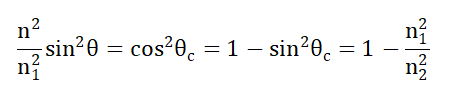Solving the above equation we get,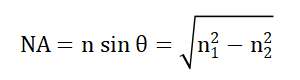This is an online calculator that calculates Numerical Aperture. Just enter the Refractive Index and the Maximal Half-Angle to get the corresponding Numerical Aperture value.

• Degrees

### Result

• Numerical Aperture

Numerical Aperture is a dimensionless quantity that determines the ability of an optical fiber to gather light. It is the measure of the range of angles over which a system can accept light or the angular acceptance of a system for the incoming light. It is given by,Where 'n' is the refractive index of the medium from which the light enters a system. It is also a dimensionless quantity that describes the change in the velocity of light when travelling through that medium.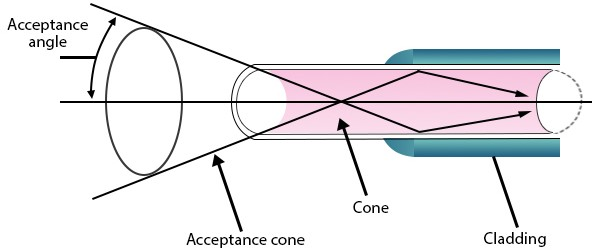Here 'θ' called the acceptance angle is the maximum incident angle at which an optical system will permit light into it.

This is an online calculator that calculates Numerical Aperture. Just enter the Refractive Index, Aperture Diameter, and Focal Length to get the corresponding Numerical Aperture value.

• mm
• mm

### Result

• Numerical Aperture

Numerical Aperture is a dimensionless quantity that determines the ability of an optical system to gather light. It is the measure of the range of angles over which a system can accept light or the angular acceptance of a system for the incoming light. It is given by,Where 'n' is the refractive index of the medium from which the light enters a system. It is also a dimensionless quantity that describes the change in the velocity of light when travelling through that medium. Here 'θ' called the acceptance angle is the maximum incident angle at which an optical system will permit light into it.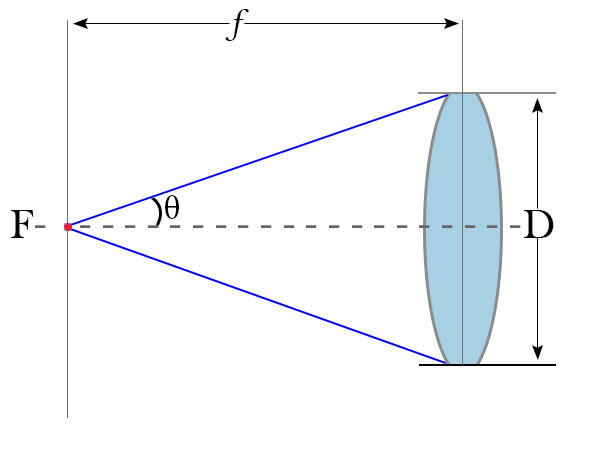Now consider the case of a thin lens, 'θ' is the acceptance angle, 'f' is the focal length of the lens and 'D' is the diameter of the lens.

From the above figure, applying trigonometric conventions, we get,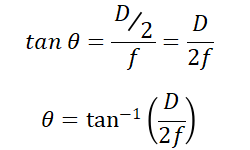So the equation for numerical aperture becomes,